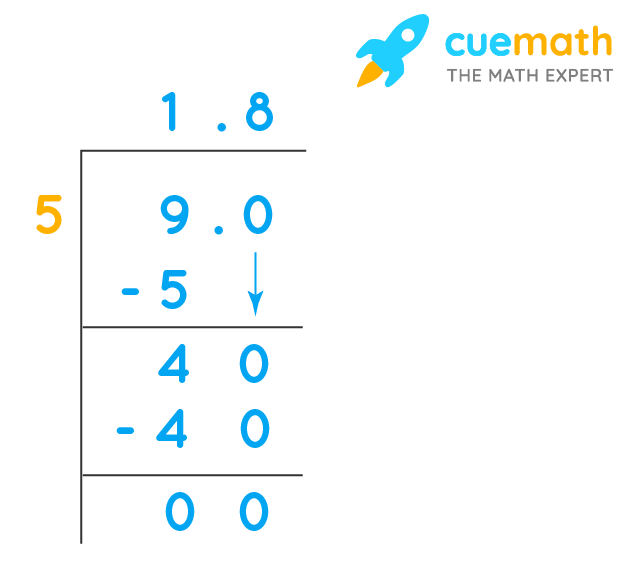# What is 9 divided by 5

## Question: What is 9 divided by 5?

The division of 9 by 5 results in a decimal number.

## Answer: 9 divided by 5 is 1.8

Let us understand this with the long division shown below.

## Explanation

When there is no common factor between numerator and denominator except 1, the quotient of the division of two numbers is always a decimal number.